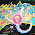Monday, February 21, 2011

Is paraconsistent arithmetic categorical?

Is naive set theory capable of describing and pinning down the intended model of arithmetic?

On the one hand, it doesn't seem very likely. With so many more sets out there to cause trouble, and easy ways to make isomorphisms fail (the totality of the ordinals isn't even isomorphic to itself, for instance), the odds of showing that any two peano relations coincide are low.

On the other hand, taking naive set theory as our base opens some intriguing possibilities. In particular, we can stay in a first order language, while at the same time, noting that the axiomatic equivalence

x is a member of X iff Xx

essentially grants us all the expressive power of second (and higher) order logic. And we know that classical second order theories can establish (classical) categoricity.

And so the question of the month is from Peter Smith's Godel book (p. 186). He's asking rhetorically, but in the paraconsistent context it's an open problem:

"If second order arithmetic does pin down the structure of the natural numbers, then -- given that any arithmetic sentence makes a determinate claim about this structure -- it apparently follows that this theory does enough to settle the truth value of every arithmetic sentence. Which makes it sound as if there can after all be a (consistent) negation-complete axiomatic theory of arithmetic..."

Well, it's an open problem if you leave out that "consistency" part...

1 comment:

1.Hello, I'm not a formally trained mathematician - just a hobbyist, but recently I constructed a proven recursive arithmetic which extends the "standard" model and it seems rather satisfying from a foundational perspective; perhaps you would find it interesting? I established set theoretic foundations in ZFC/AFA but essentially you can think of it as the structure {N x N, <, +, *, (1,0), (0,0)} with the lexicographic order, coordinate-wise addition, and multiplication defined by:

(a, b) * (c, d) = (a * c, b * c + a * d + b * d).

What’s further, when I extended this structure out to algebraic closure, it occurred to me that the relation and operations deployed could be generalized in countably many ways, leading to a countable subsumption hierarchy of recursive arithmetics bounded above by the Church-Kleene ordinal:

0) {N, <, +, *, 1, 0};

1) {N x N, <, +, *, (1, 0), (0, 0)};

2) {N x N x N x N, <, +, *, (1, 0, 0, 0), (0, 0, 0, 0)};

3) {N x N x N x N x N x N x N x N, <, +, *, (1, 0, 0, 0,0, 0, 0, 0 ), (0, 0, 0, 0, 0, 0, 0, 0)};

.

.

.

As you see, if you think of the domains as well-founded ordinals, then the exponents conform to the geometric sequence {2^n}. I hope I'm not being overly forward, but if you are, in fact, interested, then you can find the relevant papers on my Vixra profile: Wes Hansen, Vixra.

My sincere apologies if you find this distracting!